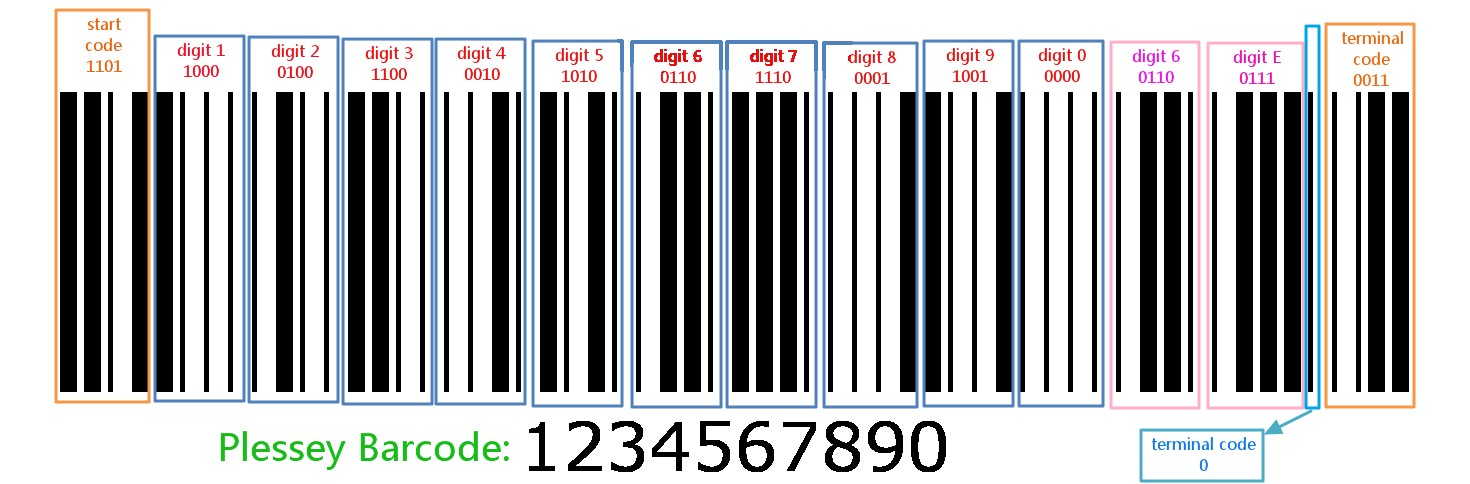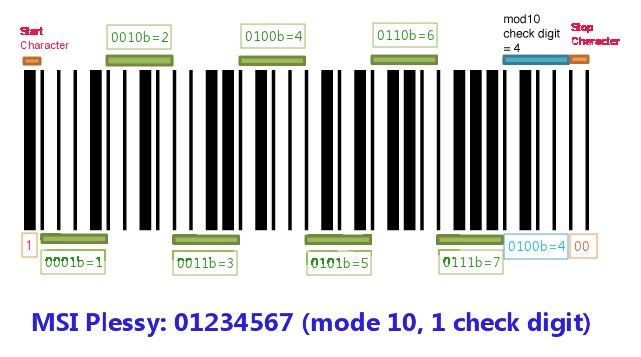# Plessey & MSI Symbology Introduction

Version:v1.1

Abstract

This article explains the details of Plessey and MSI symbology.This article provide multiple formats:
 Reading Online HTML HTMLs PDF CHM TXT RTF Download(7Zip package) HTML HTMLs PDF CHM TXT RTF

HTML version online address:

http://www.crifan.com/files/doc/docbook/symbology_plessey/release/html/symbology_plessey.html

Any question or bug report are encouraged to post in the discussion group:

http://www.crifan.com/bbs/categories/symbology_plessey/

2012-08-09

Revision History
Revision 1.0 2011-05-17 crl
1. explain the plessey and msi symboloy
Revision 1.1 2012-08-09 crl
1. Release via Docbook

List of Figures

1.1. Plessey Barcode Format Example
1.2. Plessey Barcode “01234567890” Example
2.1. MSI exapmle 01234567 mode 10

List of Tables

1.1. Plessey Barcode Format
1.2. Plessey Bits Encoding Rule
2.1. MSI Barcode Format
2.2. Mode 10 Check Digit Example
2.3. Mode 1010 Check Digit Example
2.4. Mode 11 Check Digit Example

# Chapter 1. Plessey

Abstract

## 1.1. What is Plessey

Plessey barcode/code is a 1D linear barcode symbology, based on Pulse Wide Modulation.

Plessey is originally developed in 1971 by The Plessey Company plc, a British-based company. So, sometimes is called “UK Plessey”.

So,

Plessey = UK Plessey

## 1.2. Plessey Barcode Format

### 1.2.1. An Example

Figure 1.1. Plessey Barcode Format ExampleFigure 1.2. Plessey Barcode “01234567890” Example### 1.2.2. How to represent bits/digits/characters

Plessey’s barcode pattern is so simple that, with casual familiarity with the code, you can actually read the bar codes with your eyes.

Plessey basic code rule is:

• Wide bar following by narrow space is a "1" bit.
• Narrow bar followed by wide space is a "0" bit.

And each digit number is represented by four bit.

If you only calculate the bar (horizontal black line), the wide bar means ‘1’ and the narrow bar means ‘0’.

So, the digit ‘1’:

• BCD (Binary- Coded Decimal) is 0001b
• reversed BCD is 1000b
• wide bar + narrow bar + narrow bar + narrow bar

### 1.2.3. Barcode Format

Table 1.1. Plessey Barcode Format

start character Any number of labels/characters/digits Checksum / check digit / code A stop / termination character / block / pitch bar the reverse start code
4it =1101 XXX...(eg, 012345...) 8bit(eg. 0110 0111) 1bit=X(eg, 1) 4bit=0011

In which:

• the forward start code

The forward start code is "1101". It defines the beginning of the encoded text.

• the label / data digits

The characters of the text to encode are hexadecimal values encoded as reversed BCD.

Table 1.2. Plessey Bits Encoding Rule

Value Encoding Value Encoding Value Encoding Value Encoding
0 0000 4 0010 8 0001 C 0011
1 1000 5 1010 9 1001 D 1011
2 0100 6 0110 A 0101 E 0111
3 1100 7 1110 B 1101 F 1111

• the check code, for error detection

The check code for error detection and correction is as CRC, using polynomial division.

Is uses 2 characters, or 8 bits.

The generator polynomial is g(x) = x8 + x7 + x6 + x5 + x3 + 1 with n=8, in binary "111101001".

• the termination bar

The termination bar follows the CRC. It's a full pitch bar.

• the reverse start code

The reverse start code, with the forward start code, are used to detect the direction of reading : from left to right or right to left.

It's encoded as a reversed "0011".

Reversed because the "0" is represented by a wide space and simple bar, and "1" as a simple space and wide bar.

## 1.3. Prons-and-Cons

The chief advantages are the relative ease of printing using the dot-matrix printers popular at the time of the code's introduction, and its somewhat higher density than the more common 2 of 5 and 3 of 9 codes.

## 1.4. Usage Filed

It is one of the first barcode symbology, and is still used in some rare libraries and for shelf tags in retail stores, in part as a solution to their internal requirement for stock control.

The Plessey was first used in the early 1970s by J.Sainsbury to identify all of its products on supermarket shelves for its product restocking system.

## 1.5. Variations

However, Plessey does not encode numbers efficiently, and other characteristics of the language make it one of the most error-prone of all bar code languages.

Accordingly, the MSI company came out with a revised standard for the Plessey language (now called MSI Plessey, also known as Plessey modified) which employed a second check digit. This improved the reliability of the language enough so that the MSI version of the code has continued in use in specialty applications.

Except the MSI, several other variations of Plessey is Anker Code by ADS Company, Telxon. It is difficult to have the specifications for them nowadays and thus hard to tell the differences between them (except for MSI), because is was mainly available as paper document and in discontinued since then.

# Chapter 2. MSI Plessey

Abstract

## 2.1. What is MSI Plessey

MSI Plessey is a barcode symbology, is a variation of Plessey, is the most widely supported variation of Plessey.

Also called “Modified Plessey”, or MSI.

So:

MSI = MSI Plessey = Modified Plessey

Following description will use MSI.

MSI was developed by the MSI Data Corporation, based on the original Plessey Code, Currently maintained by Symbol Technologies, Inc.

While the length of an MSI bar code can be of any length, a given application usually implements a fixed-length code

## 2.2. Characteristics

MSI is a continuous symbology that is not self-checking, based on Pulse-Width Modulation

### 2.2.1. Character set

The MSI character set consist of the numbers 0..9 as well as two "guard" characters (representing the beginning and end of the barcode).

So, like Interleaved 2-of-5 and UPC, MSI is an all-numeric language, not support alphabetic characters.

### 2.2.2. Coding Rule

The bit representation is same with Plessey:

• Wide bar following by narrow space is a "1" bit.
• Narrow bar followed by wide space is a "0" bit.

But the digit representation is more simple:

Each digit number is represented by four bit.

Except the start and stop bits, If you only calculate the bar (horizontal black line), the wide bar means ‘1’ and the narrow bar means ‘0’,

then it is the same rule as using binary to represent the digit:

Digit 0 = 0000b

Digit 1 = 0001b

Digit 2 = 0010b

Digit 3 = 0011b

...

## 2.3. MSI Barcode Example

Figure 2.1. MSI exapmle 01234567 mode 10## 2.4. MSI Barcode Format

Table 2.1. MSI Barcode Format

Format start character Any number of digits Checksum digit(s) A stop character
Explanation a wide bar followed by a narrow space Digits 5 modes:
• No check digit (least common)
• Mod 10 (most common)
• Mod 11
• Mod 1010
• Mod 1110
narrow bar, a wide space, then a narrow bar
Digits/Bits Bit: 1 Digits: XXX... Digit(s): X/XX Bits: 00

### 2.4.1. Checksum digit(s)

The MSI barcode uses one of five possible schemes for calculating a check digit:

• No check digit (least common)
• Mod 10 (most common)
• Mod 11
• Mod 1010
• Mod 1110

#### 2.4.1.1. Mode 10 and Mode 1010

The checksum algorithm for Mode 10 is to use “Luhn algorithm”:

1. Counting from the check digit, which is the rightmost one, and moving left, double (x 2) the value of every second digit.
2. Sum the digits of the products (eg, 10 ⇒ 1+0 = 1, 14 ⇒ 1+ 4 =5) together with the un-doubled digits from the original number
3. Got the sum, then do: sum Mod 10 = check digit

Assume an example of an account number "1234567" that will have a check digit added, making it of the form “1234567X”:

Then the steps to calculate the X are:

Table 2.2. Mode 10 Check Digit Example

Example 1 2 3 4 5 6 7 Mode 10 Check digit
(1) Append X 1 2 3 4 5 6 7 X
(2) From rightmost, every second digit × 2 2   6   10   14
(3) List all 2 2 6 4 1+0 6 1+4
(4) Got Summary Sum=2+2+6+4+1+0+6+1+4=26, 26 Mod 10 = 4 X = 4
(5)Result 12345674

For the second checksum digit, the calculation method is still the same, just take the “12345674” as the input number, the calculate the X for “12345674X

##### 2.4.1.1.1. Mode 1010 Example

Table 2.3. Mode 1010 Check Digit Example

Example 1 2 3 4 5 6 7 4 Mode 1010 Check digit
(1) Append X 1 2 3 4 5 6 7 4 X
(2) From rightmost, every second digit × 2   4   8   12   8
(3) List all 1 4 3 8 5 1+2 7 8
(4) Got Summary Sum=1+4+3+8+5+1+2+7+8=39, 39 Mod 10 = 1 X = 1
(5)Result 123456741

#### 2.4.1.2. Mode 11

To calculate the Modulo 11 check digit, use the following process:

1. Assign a weight to each character in the code, starting with a weight of 2 in the right-most position and incrementing by one as you move to the left. After you reach a weight of 7, the next digit will have a weight of 2 (that is, weighting goes from 2 to 7 and then wraps around back to 2)
2. Multiply the value of each character by its weight, and sum the result of all the characters
3. Perform a modulo 11 on the result (sum) of step 2

Assume an example of an account number "1234567" that will have a mode 11 check digit added, making it of the form “1234567X”:

Table 2.4. Mode 11 Check Digit Example

Example 1 2 3 4 5 6 7 Mode 11 Check digit
(1) Add weight 2 7 6 5 4 3 2 X
(2) digit × weight 2 14 18 20 20 18 14
(3)add all, get sum Sum=2+14+18+20+20+18+14=106, 106 Mod 11 = 4 X = 4
(4)Result 12345674

And for the mode 1110, take “1234567”as example, just to use the mode 11 for “1234567”to calculate the first mode 11 check digit, is 4, then do mode 10 for “12345674”then calculate out the second mode 10 check digit, is 1, so the whole result is “123456741

TipWhile most MSI barcodes include at least one checksum digit, so in many case, the printed MSI barcode does not show out the first checksum digit, while only show the second checksum digit if exists.

## 2.5. Usage Filed

MSI is used primarily for inventory control, marking storage containers and shelves in warehouse environments.

MSI, and other symbologies based on Pulse-Width Modulation, offer no significant benefit over more modern symbologies. While it is not a bad idea to support MSI for legacy bar codes, most new applications do not choose MSI as their symbology of choice.

 Plessey